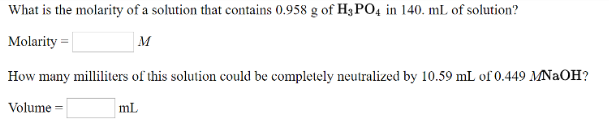# Problem: What is the molarity of a solution that contains 0.958 g of H 3PO4 in 140. mL of solution? How many milliliters of this solution could be completely neutralized by 10.59 mL of 0.449 M NaOH?

###### FREE Expert Solution
95% (274 ratings)###### Problem Details

What is the molarity of a solution that contains 0.958 g of H 3POin 140. mL of solution?

How many milliliters of this solution could be completely neutralized by 10.59 mL of 0.449 M NaOH?